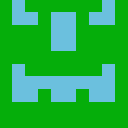# Odoo Aide

4 Answers

Dear Silviaa,

According my knowledge , One2many is a virtual field.Since it is It must have a Many2one model behind.So that it,s data can be linked to the current record on the self.

for eg:

`class Target(models.Model):    _name = 'projection.target'    _rec_name = 'sales_man'    _sql_constraints = [('sales_man', 'UNIQUE(sales_man)', 'The employee has already assigned to a target'), ]    sales_man = fields.Many2one('res.users', string="Select Employee", required=True)    working_days = fields.Integer(compute="computewkng")    target = fields.Char(string='Total Projection', readonly=True, compute='totalprojection')    sal = fields.Float(string='Achievement', compute="calculatetotalsales", readonly=True)    average = fields.Float(String='Average', compute='computeavg')    variance = fields.Float(string='Variance', compute='computevariance')    data = fields.One2many('projection.onetomanydata', 'inv', ondelete='cascade') #this model has a One2many field that is linked with the Many2one on another moduleclass OneToManyData(models.Model):    _name = 'projection.onetomanydata'    _rec_name = 'name'    _sql_constraints = [('date', 'UNIQUE(date)', 'Employee Has Already Assigned to a Target on This date'), ]    name = fields.Char(default='Daily Projection', invisible=True)    date = fields.Date(string="Date", required=True)    target = fields.Integer(string="Daily Projection", required=True)    inv = fields.Many2one('projection.target')#here the inv links this model to the first one#its just a matter of 2 lines of code.`

one2many field attributes, comodel_name, and inverse_name (i.e. foreign key) are mandatory except in the case of related fields or field extensions.

In your case, you can display all records in wizard on button click...
###### Writer1 Abonné(e)s
###### About This Community

This platform is for beginners and experts willing to share their Odoo knowledge. It's not a forum to discuss ideas, but a knowledge base of questions and their answers.

###### Odoo Training Center

Access to our E-learning platform and experience all Odoo Apps through learning videos, exercises and Quizz.